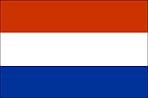Welcome to the tablet and desktop friendly page. Here you'll find free educational games aimed at children between 5 and 8 years old. Here are some help videos to get you started. The games are linked to the UK KS 1 and 2 curricula.

Apps (to buy)
Teaching Tools
PrintablesNumber - number and place valuePlace valuePlace ValuePlace ValuePlace ValuePlace ValueCounting & Ordering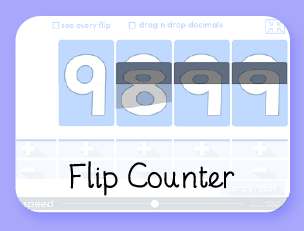Counting / Decimals / +10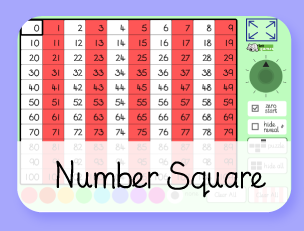Various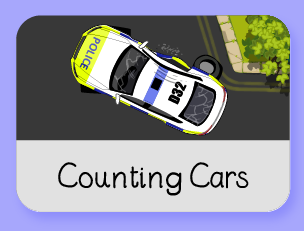CountingCountingCounting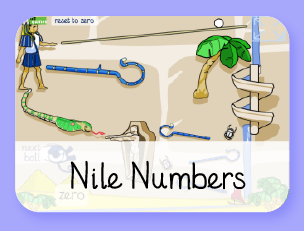Number Spelling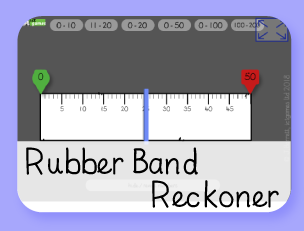Ordering Numbers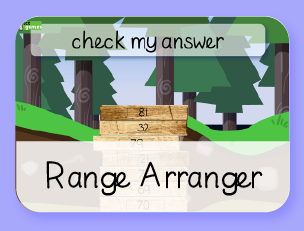Ordering numbers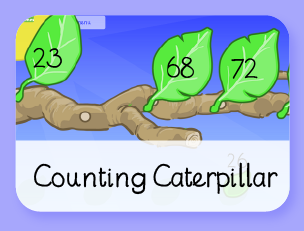Ordering numbersOdds & Evens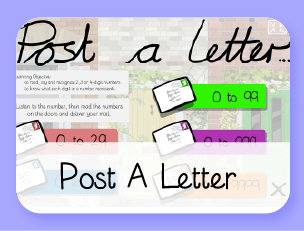Reading numbers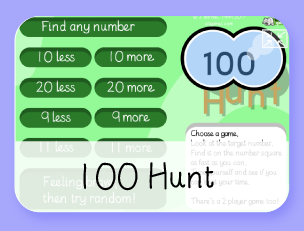+/- 10, 9, 11
Number - addition & subtraction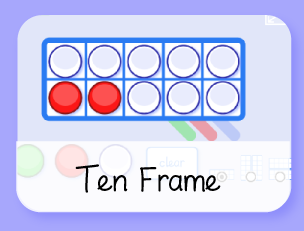Number Facts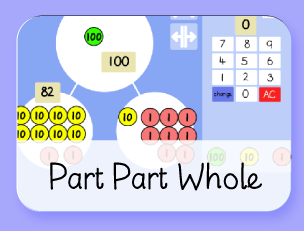Number FactsSubtraction+/- 10, 9, 11Missing Numbers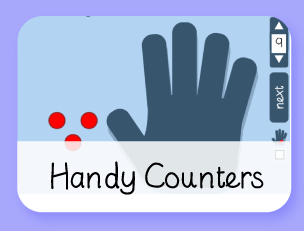Subtraction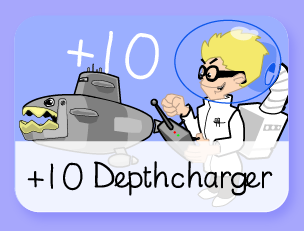Adding 10Addition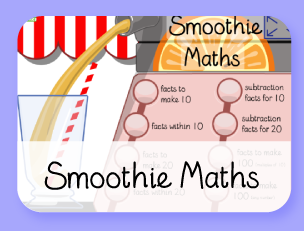Number FactsBonds of 10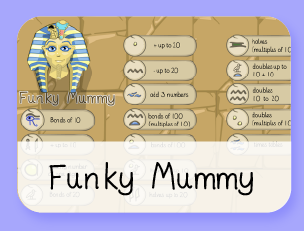Number facts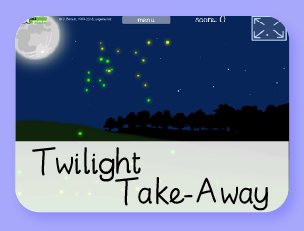Subtraction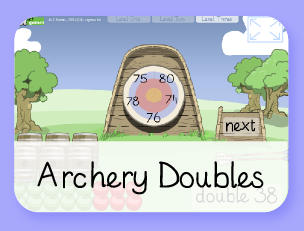Doubles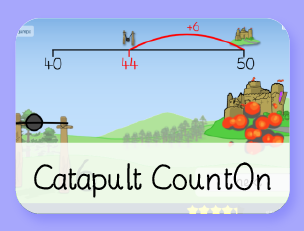Bridging to 10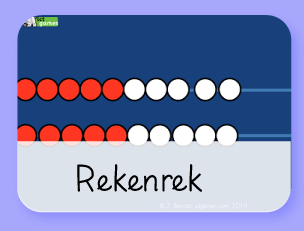Addition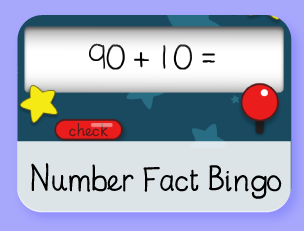+/-/x Facts
Number - multiplication & divisionCounting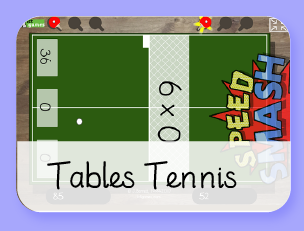Times tables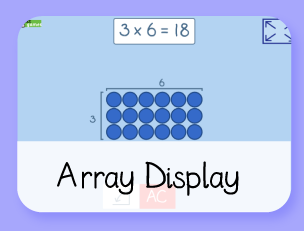Multiplication & Division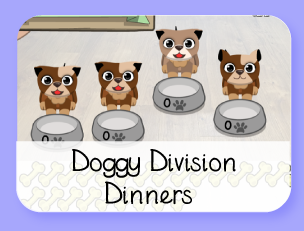DivisionNumber facts
Number - fractionsFractionsFractions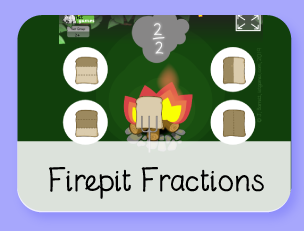Fractions
Measurement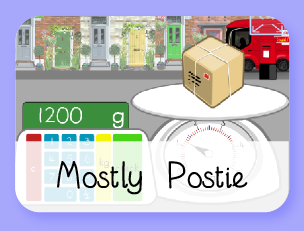Measure(g/kg)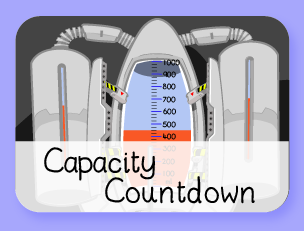Measure(ml/l)Clock DemonstratorTime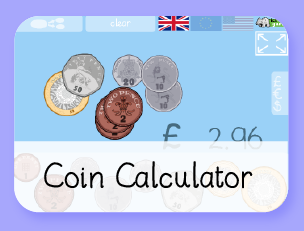MoneyMoney
Geometry – properties of shapes
VariousThe Date Chart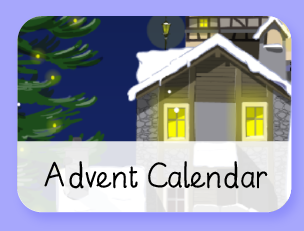Christmas